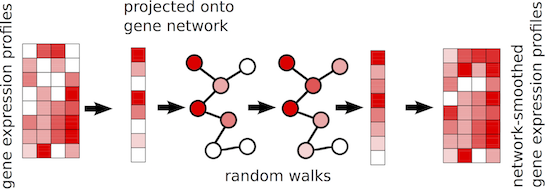# 1 Introduction

netSmooth implements a network-smoothing framework to smooth single-cell gene expression data as well as other omics datasets. The algorithm is a graph based diffusion process on networks. The intuition behind the algorithm is that gene networks encoding coexpression patterns may be used to smooth scRNA-seq expression data, since the gene expression values of connected nodes in the network will be predictive of each other. Protein-protein interaction (PPI) networks and coexpression networks are among the networks that could be used for such procedure.

More precisely, netSmooth works as follows. First, the gene expression values or other quantitative values per gene from each sample is projected on to the provided network. Then, the diffusion process is used to smooth the expression values of adjacent genes in the graph, so that a genes expression value represent an estimate of expression levels based the gene it self, as well as the expression values of the neighbors in the graph. The rate at which expression values of genes diffuse to their neighbors is degree-normalized, so that genes with many edges will affect their neighbors less than genes with more specific interactions. The implementation has one free parameter, `alpha`, which controls if the diffusion will be local or will reach further in the graph. Higher the value, the further the diffusion will reach. The netSmooth package implements strategies to optimize the value of `alpha`.Figure 1: Network-smoothing concept

In summary, netSmooth enables users to smooth quantitative values associated with genes using a gene interaction network such as a protein-protein interaction network. The following sections of this vignette demonstrate functionality of `netSmooth` package.

# 2 Smoothing single-cell gene expression data with netSmooth() function

The workhorse of the netSmooth package is the `netSmooth()` function. This function takes at least two arguments, a network and genes-by-samples matrix as input, and performs smoothing on genes-by-samples matrix. The network should be organized as an adjacency matrix and its row and column names should match the row names of genes-by-samples matrix.

We will demonstrate the usage of the `netSmooth()` function using a subset of human PPI and a subset of single-cell RNA-seq data from GSE44183-GPL11154. We will first load the example datasets that are available through netSmooth package.

``````data(smallPPI)
data(smallscRNAseq)``````

We can now smooth the gene expression network now with `netSmooth()` function. We will use `alpha=0.5`.

``smallscRNAseq.sm.se <- netSmooth(smallscRNAseq, smallPPI, alpha=0.5)``
``## Using given alpha: 0.5``
``````smallscRNAseq.sm.sce <- SingleCellExperiment(
assays=list(counts=assay(smallscRNAseq.sm.se)),
colData=colData(smallscRNAseq.sm.se)
)``````

Now, we can look at the smoothed and raw expression values using a heatmap.

``````anno.df <- data.frame(cell.type=colData(smallscRNAseq)\$source_name_ch1)
rownames(anno.df) <- colnames(smallscRNAseq)
pheatmap(log2(assay(smallscRNAseq)+1), annotation_col = anno.df,
show_rownames = FALSE, show_colnames = FALSE,
main="before netSmooth")``````